Techist - Tech Forum - View Single Post - Percentage Button

 Thread: Percentage Button View Single Post11-13-2009, 04:16 AM   #1 (permalink)
Oreo
-Deactivated-

Join Date: Dec 2006
Location: England
Posts: 5,723Percentage Button

I can not figure out how to do the percentage button, if someone could show me how to do it (using the same kind of code i've already used, not to complex) that would be greatIt's all done in VB6, and obviously there all actually seperated by whatever button each chunk of code works with. Sorry if it looks more confusing than it actually is !

Basically i have all the number buttons from 0 to 9, the +, - , divide, sqaure root, answer, clear and period button all working together (well the decimal button will only work to 1 decimal place) We have to recreate the Microsoft Windows Calculator for school work, so it's gotta work very similar to that.

Here is my current code.. it's kinda long.

Quote:
 Dim b As Integer Dim c As Integer Dim a As Integer Dim Number As Double Dim Number2 As Double Dim Number3 As Double Dim Number4 As Double Dim Number5 As Double Dim Number6 As Double Dim Number7 As Double Dim Number8 As Double Dim Number9 As Double Dim Number10 As Integer Dim Number11 As Integer Dim Number12 As Integer Dim Number13 As Integer Dim Number14 As Integer Dim Number15 As Integer Dim d As Integer 'Imports System.Math Dim e As Integer Dim f As Integer Dim g As Integer Private Sub ans_Click() If (a = 2) Then Number2 = Text1 Text1 = Number + Number2 ElseIf (a = 1) Then Number4 = Text1 Text1 = Number3 - Number4 ElseIf (a = 3) Then Number6 = Text1 Text1 = Number5 * Number6 ElseIf (a = 4) Then Number8 = Text1 Text1 = Number7 / Number8 ElseIf (e = 1) Then Number10 = Text1 Text1 = Math.Sqr(Number10) ElseIf (f = 1) Then Number11 = Text1 End If End Sub Private Sub Command1_Click() Number = 0 Number2 = 0 Number3 = 0 Number4 = 0 Number5 = 0 Number6 = 0 Number7 = 0 Number8 = 0 Text1 = "0." End Sub Private Sub Command2_Click() If Text1 = "0." Then Text1 = "." Else x = Len(Text1) temp = Left(Text1, x + 1) Text1 = temp + "." End If End Sub Private Sub divide_Click() a = 4 Number7 = Text1 Text1 = 0 End Sub Private Sub eight_Click() If Text1 = "0." Then Text1 = "8." ElseIf (d = 0) Then x = Len(Text1) temp = Left(Text1, x - 1) Text1 = temp + "8" + "." ElseIf (d = 1) Then x = Len(Text1) temp = Left(Text1, x - 1) Text1 = temp + "." + "8" d = 0 End If End Sub Private Sub five_Click() If Text1 = "0." Then Text1 = "5." ElseIf (d = 0) Then x = Len(Text1) temp = Left(Text1, x - 1) Text1 = temp + "5" + "." ElseIf (d = 1) Then x = Len(Text1) temp = Left(Text1, x - 1) Text1 = temp + "." + "5" d = 0 End If End Sub Private Sub four_Click() If Text1 = "0." Then Text1 = "4." ElseIf (d = 0) Then x = Len(Text1) temp = Left(Text1, x - 1) Text1 = temp + "4" + "." ElseIf (d = 1) Then x = Len(Text1) temp = Left(Text1, x - 1) Text1 = temp + "." + "4" d = 0 End If End Sub Private Sub minus_Click() a = 1 Number3 = Text1 Text1 = 0 End Sub Private Sub nine_Click() If Text1 = "0." Then Text1 = "9." ElseIf (d = 0) Then x = Len(Text1) temp = Left(Text1, x - 1) Text1 = temp + "9" + "." ElseIf (d = 1) Then x = Len(Text1) temp = Left(Text1, x - 1) Text1 = temp + "." + "9" d = 0 End If End Sub Private Sub one_Click() If Text1 = "0." Then Text1 = "1." ElseIf (d = 0) Then x = Len(Text1) temp = Left(Text1, x - 1) Text1 = temp + "1" + "." ElseIf (d = 1) Then x = Len(Text1) temp = Left(Text1, x - 1) Text1 = temp + "." + "1" d = 0 End If End Sub Private Sub perc_Click() Number12 = Text1 Text1 = "0." Number13 = Number12 End Sub Private Sub plus_Click() a = 2 Number = Text1 Text1 = 0 End Sub Private Sub point_Click() d = 1 End Sub Private Sub seven_Click() If Text1 = "0." Then Text1 = "7." ElseIf (d = 0) Then x = Len(Text1) temp = Left(Text1, x - 1) Text1 = temp + "7" + "." ElseIf (d = 1) Then x = Len(Text1) temp = Left(Text1, x - 1) Text1 = temp + "." + "7" d = 0 End If End Sub Private Sub six_Click() If Text1 = "0." Then Text1 = "6." ElseIf (d = 0) Then x = Len(Text1) temp = Left(Text1, x - 1) Text1 = temp + "6" + "." ElseIf (d = 1) Then x = Len(Text1) temp = Left(Text1, x - 1) Text1 = temp + "." + "6" d = 0 End If End Sub Private Sub sqrt_Click() e = 1 End Sub Private Sub three_Click() If Text1 = "0." Then Text1 = "3." ElseIf (d = 0) Then x = Len(Text1) temp = Left(Text1, x - 1) Text1 = temp + "3" + "." ElseIf (d = 1) Then x = Len(Text1) temp = Left(Text1, x - 1) Text1 = temp + "." + "3" d = 0 End If End Sub Private Sub times_Click() a = 3 Number5 = Text1 Text1 = 0 End Sub Private Sub two_Click() If Text1 = "0." Then Text1 = "2." ElseIf (d = 0) Then x = Len(Text1) temp = Left(Text1, x - 1) Text1 = temp + "2" + "." ElseIf (d = 1) Then x = Len(Text1) temp = Left(Text1, x - 1) Text1 = temp + "." + "2" d = 0 End If End Sub Private Sub zero_Click() If Text1 = "0." Then Text1 = "0." ElseIf (d = 0) Then x = Len(Text1) temp = Left(Text1, x - 1) Text1 = temp + "0" + "." ElseIf (d = 1) Then x = Len(Text1) temp = Left(Text1, x - 1) Text1 = temp + "0" + "." d = 0 End If End Sub
__________________» Recent Threads

•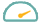Fuelly
•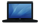Techist
•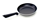Cooking Forum
•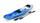Kayaking & Rafting Forum
•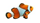Aquarium Forum
•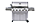BBQ Forum
•Early Retirement
•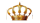Royal Forums
•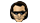U2 Music Forum
•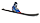Ski Forum
•Computer Forums
•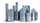CityProfile Local Forum

Our Communities

Our communities encompass many different hobbies and interests, but each one is built on friendly, intelligent membership.

•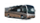iRV2 RV Forum
•Airstream Trailer Forum
•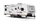Forest River Forums
•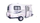Fiberglass RV Forums
•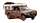Wander The West
•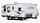Jayco RV Forum
•Luxury Coach Forum
•Escape Trailer Forum
•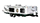Hilo Trailer Forum
•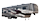5th Wheel Forums
•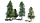RV Park Reviews
•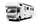Truck Conversions
•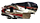Redwood Owners
•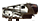Crossroads Owners
•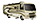Thor RV Forum
•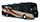Winnebago RV Forum
•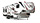Dutchmen RV Forum
•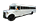School Bus Conversions
•Sunline Trailers Forum
•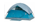Campground Reviews
•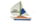Cruisers Sailing Forum
•Rigid Inflatable Boats
•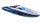Performance Boat Forum
•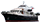Trawler Boating Forums
•Maxum Owners Club
•Sail Blogs
•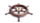Sailing Wiki
•Cruisers Log
•Yacht Blogs

Marine Communities

Our Marine websites focus on Cruising and Sailing Vessels, including forums and the largest cruising Wiki project on the web today.

» More about our Marine Communities